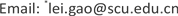1. 引言

Maxworth   通过活塞装置产生涡环并研究了其生成与演化的过程。Didden  建立了Slug模型描述了射流产生的涡环的物理特征。Shariff等  和Lim等  对之前的在静止、非分层环境中涡环的动力学特征进行了总结。Gharib等  通过对活塞–圆管装置产生的轴对称涡环的形成过程进行研究，发现了涡环

F r = R i – 1 R i = D Δ ρ ρ g / U p 2 (1)

2. 实验部分

Experiment parameter
caseD(m)U0(m/s)L(m)ρ(kg/m3)ρ0(kg/m3)Δρ/ρ0(%)Ri
H10.020.060.04998.1998.100
H20.020.060.06998.1998.100
N10.020.060.04996.9997.8−0.09−0.050
N20.020.060.06996.7997.6−0.09−0.050
N30.020.060.04996.4998.1−0.17−0.098
N40.020.060.06996.0997.8−0.18−0.102
N50.020.060.04994.0997.7−0.37−0.201
N60.020.060.06993.9997.5−0.36−0.199
N70.020.060.04992.0997.6−0.56−0.304
N80.020.060.06992.0997.5−0.55−0.298

3. 结果与分析

3.1. 涡量场与速度场

3.2. 涡核位置与涡环半径

3.3. 涡环的环量、冲量与速度

I = π ∫ ​ ω r 2 d x d r (2)

Γ = ∫ ​ ω r 2 d x d r (3)

Γ ′ = U p 2 t / 2 (4)

I = ρ π R 2 (5)

V = Γ 4 π R ( ln 8 ε − 1 4 ) (6)

4. 结语